﻿ 计及风沙磨损的架空输电线路冰风荷载失效概率评估方法 Failure Probability Assessment Method for Overhead Transmission Lines under Ice and Wind Loads considering Blowing Sand Wear

Smart Grid
Vol. 09  No. 03 ( 2019 ), Article ID: 30604 , 11 pages
10.12677/SG.2019.93011

Failure Probability Assessment Method for Overhead Transmission Lines under Ice and Wind Loads considering Blowing Sand Wear

Zeqing Liu1, Xin Li1, Xiaofu Xiong1, Wenbing Zhuang2

1School of Electrical Engineering, Chongqing University, Chongqing

2Electric Power Research Institute of State Grid Xinjiang Electric Power Co. Ltd., Urumqi Xinjiang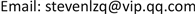Received: May 10th, 2019; accepted: May 27th, 2019; published: Jun. 3rd, 2019ABSTRACT

The transmission corridor in Xinjiang is exposed to a severe climate; the strength of the overhead line will be lost after being subjected to blowing sand wear. Especially in the freezing weather, the wind excitation plus icing is prone to structural failure of the overhead line. It is of great significance to study the failure probability evaluation method of the overhead line under ice and wind loads considering blowing sand wear. In this paper, the ice load is calculated according to the ice thickness prediction model based on the micro-meteorological monitoring devices. Then, according to the strength interference theory, the failure probability of the transmission line is dynamically evaluated by comparing the residual strength of the transmission line after blowing sand wear and the ice and wind combination loads. Finally, the feasibility and effectiveness of the proposed method are verified through the inversion analysis of an actual case in Xinjiang. The research results are helpful to check the operation status of the transmission line timely and prevent the failure caused by the line icing and strong wind.

1重庆大学电气工程学院，重庆

2国网新疆电力有限公司电力科学研究院，新疆 乌鲁木齐1. 引言

1998年美国、加拿大发生严重冰灾，加拿大东南部和魁北克南部省份的受灾情况最为严重，造成了28人死亡，470万加拿大人和50万美国人遭受停电影响  ；2008年，我国南方大范围遭遇了严重的持续低温雨雪冰冻灾害天气，大范围雨雪冰冻天气影响了我国19个省区，造成大范围倒塔、断线和闪络事故，贵州、湖南、江西和浙江省电网受损最为严重 。2018年1~2月，中国华南和华东地区普遍出现大面积的积雪冰冻天气，输电线路再次面临考验 。

2. 基于微气象监测的输电线路冰风荷载预测

2.1. 输电线路冰厚预测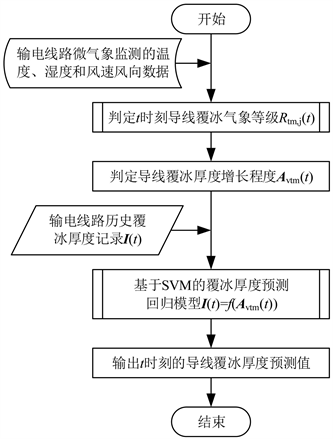Figure 1. Flowchart of conductor ice thickness prediction basedon micrometeorological monitoring data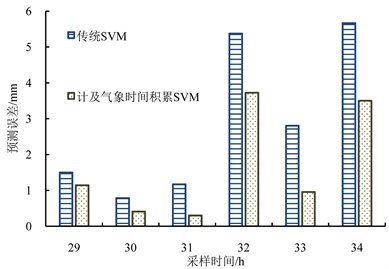Figure 2. Absolute errors of 2 forecasting models

2.2. 输电线路冰风组合荷载

${m}_{\text{ice}}=0.5\text{π}{\rho }_{\text{ice}}\Delta r\cdot {r}_{\text{ice}}$ (1)

${r}_{{}^{\text{ice}}}=0.5\Delta r+0.25\text{π}d$ (2)

${q}_{m}=\frac{9.8ac\left(d+2\Delta r\right){v}^{2}}{16}$ (3)

$H-{H}_{0}=\frac{EA{l}^{2}}{24}\left(\frac{{q}^{2}}{{H}^{2}}-\frac{{q}_{0}^{2}}{{H}_{0}^{2}}\right)$ (4)

3. 风沙磨损导致的强度损失

${W}_{\text{sand}}=0.4585{t}^{2}-11.6t+114.4$ (5)

$W=0.2712{t}^{2}-7.257t+107.9$ (6)

4. 输电线路冰风荷载失效概率评估

4.1. 失效概率模型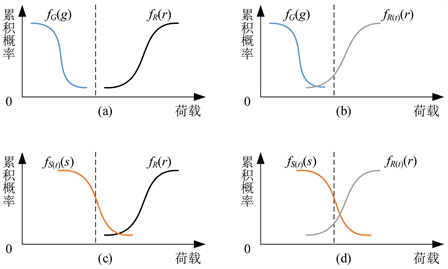Figure 3. Interferogram of strength and loadcumulative probability density curves of overhead lines

$Z\left(t\right)=g\left(R\left({t}_{1}\right),S\left({t}_{2}\right)\right)=R\left({t}_{1}\right)-S\left({t}_{2}\right)$ (7)

${P}_{f}\left({t}_{1},{t}_{2}\right)=P\left(Z\left({t}_{1},{t}_{2}\right)<0\right)=P\left(R\left({t}_{1}\right)-S\left({t}_{2}\right)<0\right)$ (8)

${f}_{R\left({t}_{1}\right),S\left({t}_{2}\right)}\left(r,s\right)={f}_{R\left({t}_{1}\right)}\left(r\right)\cdot {f}_{S\left({t}_{2}\right)}\left(s\right)$ (9)

$\begin{array}{c}{p}_{f}\left({t}_{1},{t}_{2}\right)=p\left(R\left({t}_{1}\right) (10)

${f}_{Z\left({t}_{1},{t}_{2}\right)}=\frac{1}{\sqrt{2\text{π}}{\sigma }_{Z}}\mathrm{exp}\left(-\frac{{\left(z-{\mu }_{Z\left({t}_{1},{t}_{2}\right)}\right)}^{2}}{2{\sigma }_{Z}^{2}}\right)$ (11)

 (12)

$\alpha \left({t}_{1},{t}_{2}\right)=\frac{z\left({t}_{1},{t}_{2}\right)-{\mu }_{Z\left({t}_{1},{t}_{2}\right)}}{{\sigma }_{Z}}$ (13)

${p}_{f}\left({t}_{1},{t}_{2}\right)=p\left(Z\left({t}_{1},{t}_{2}\right)<0\right)=\underset{-\infty }{\overset{-\frac{{\mu }_{z\left({t}_{1},{t}_{2}\right)}}{{\sigma }_{Z}}}{\int }}\frac{1}{\sqrt{2\text{π}}}\mathrm{exp}\left(-\frac{{\alpha }^{2}\left({t}_{1},{t}_{2}\right)}{2}\right)\text{d}\alpha$ (14)

${p}_{f}\left({t}_{1},{t}_{2}\right)=\Phi \left(-\frac{{\mu }_{z\left({t}_{1},{t}_{2}\right)}}{{\sigma }_{Z}}\right)$ (15)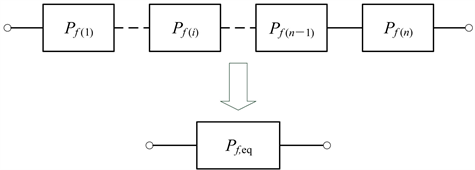Figure 4. Series equvilent modeloftransmission line failure probability

${P}_{f,\text{eq}}\left(t\right)=1-\underset{i=1}{\overset{n}{\prod }}\left(1-{P}_{f\left(i\right)}\right)$ (16)

4.2. 评估流程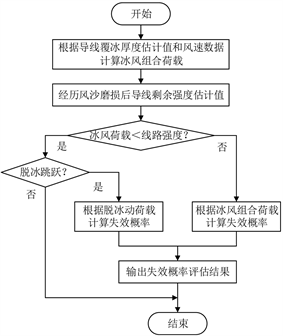Figure 5. Flowchart of transmission line failure probability evaluation

5. 算例分析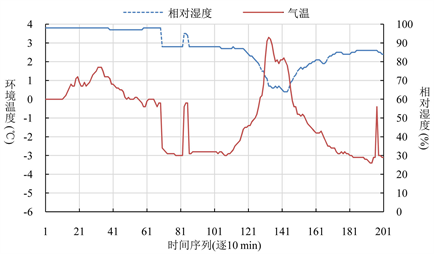Figure 6. Ambient temperature and humidity data acquired by a micrometeorological monitoring equipment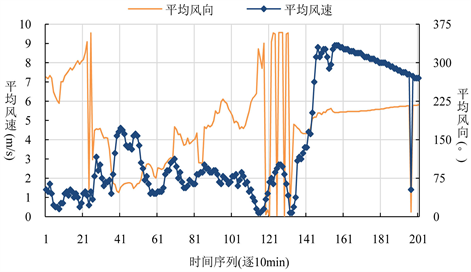Figure 7. Wind speed and wind direction data acquired by a micrometeorological monitoring equipmentFigure 8. Comparison of wind-sand wear strength loss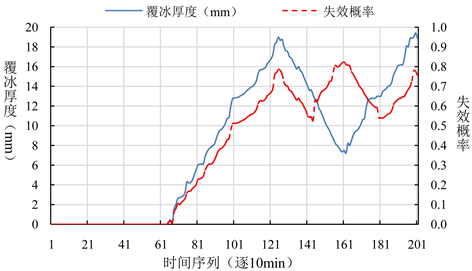Figure 9. Ice thickness and failure probability prediction

6. 结语

Failure Probability Assessment Method for Overhead Transmission Lines under Ice and Wind Loads considering Blowing Sand Wear[J]. 智能电网, 2019, 09(03): 101-111. https://doi.org/10.12677/SG.2019.93011

1. 1. 丁尧, 熊小伏, 陈强. 连续高温天气下架空线路动态载流能力评估与运行温度安全告警[J]. 智能电网, 2018, 8(5): 419-431.

2. 2. 赵渊, 魏亚楠, 范飞. 计及微振磨损与风雨荷载的输电线可靠性建模[J]. 电力系统保护与控制, 2015, 43(2): 19-25.

3. 3. 王覃梅, 孟繁荣, 毕胜. 一种用于融化输电线路初期覆冰的方法[J]. 智能电网, 2017, 5(6): 573-578.

4. 4. Koval, S.B., et al. (2006) Modeling Severe Weather Related High Voltage Transmission Line Forced Outages. Transmission & Distribution Conference & Exhibition, Dallas, 21-24 May 2006, 788-793.

5. 5. 李帅, 梁允, 李哲, 等. 基于数值天气预报结果的输电线路舞动预测[J]. 智能电网, 2016, 4(12): 1242-1246.

6. 6. 程正刚. 电力应急体系的脆弱性研究[D]: [硕士学位论文]. 上海: 上海交通大学, 2010.

7. 7. Ruszczak, B. and Tomaszewski, M. (2015) Extreme Value Analysis of Wet Snow Loads on Power Lines. IEEE Transac-tions on Power Systems, 30, 457-462.
https://doi.org/10.1109/TPWRS.2014.2321008

8. 8. 陆佳政, 蒋正龙, 雷红才, 等. 湖南电网2008年冰灾事故分析[J]. 电力系统自动化, 2008, 32(11): 16-19.

9. 9. 蒋兴良, 张志劲, 胡琴, 等. 再次面临电网冰雪灾害的反思与思考[J]. 高电压技术, 2018, 44(2): 463-469.

10. 10. 张松海, 施心陵, 李鹏, 等. 基于动态拉力与倾角的输电线路覆冰过程辨识与建模[J]. 电力系统保护与控制, 2016, 44(9): 57-61.

11. 11. 胡琴, 于洪杰, 徐勋建, 等. 分裂导线覆冰扭转特性分析及等值覆冰厚度计算[J]. 电网技术, 2016, 40(11): 3615-3620.

12. 12. 林刚, 王波, 彭辉, 等. 基于强泛化卷积神经网络的输电线路图像覆冰厚度辨识[J]. 中国电机工程学报, 2018, 38(11): 3393-3401.

13. 13. Hu, Z., He, T., Zeng, Y., et al. (2018) Fast Image Recognition of Transmission Tower Based on Big Data. Protection and Control of Modern Power Systems, 3, 149-158.
https://doi.org/10.1186/s41601-018-0088-y

14. 14. 黄新波, 孙钦东, 张冠军, 等. 线路覆冰与局部气象因素的关系[J]. 高压电器, 2008, 44(4): 289-294.

15. 15. Farzaneh, M. and Savadjiev, K. (2005) Statistical Analysis of Field Data for Precipitation Icing Accretion on Overhead Power Lines. IEEE Transactions on Power Delivery, 20, 1080-1087.
https://doi.org/10.1109/TPWRD.2004.838518

16. 16. 阳林, 郝艳捧, 黎卫国, 等. 输电线路覆冰与导线温度和微气象参数关联分析[J]. 高电压技术, 2010, 36(3): 775-781.

17. 17. 蒋兴良, 易辉. 输电线路覆冰及防护[M]. 北京: 中国电力出版社, 2001.

18. 18. 朱永灿, 黄新波, 贾建援, 等. 输电导线覆冰生长及影响因素数值分析模型[J]. 西安交通大学学报, 2015, 49(7): 120-125.

19. 19. Savadjiev, K. and Farzaneh, M. (2004) Modeling of Icing and Ice Shedding on Overhead Power Lines Based on Sta-tistical Analysis of Meteorological Data. IEEE Transactions on Power Delivery, 19, 715-721.
https://doi.org/10.1109/TPWRD.2003.822527

20. 20. Savory, E., Parke, G.A.R., Disney, P., et al. (2008) Wind-Induced Trans-mission Tower Foundation Loads: A Field Study—Design Code Comparison. Journal of Wind Engineering and Industrial Aerody-namics, 96, 1103-1110.
https://doi.org/10.1016/j.jweia.2007.06.033

21. 21. Yi, H., et al. (2007) Parameters for Wind Caused Overhead Transmission Line Swing and Fault. IEEE Region 10 Conference, Hong Kong, 14-17 November 2006, 1-4.
https://doi.org/10.1109/TENCON.2006.343973

22. 22. 谢强, 张勇, 李杰. 华东电网500 kV任上5237线飑线风致倒塔事故调查分析[J]. 电网技术, 2006, 30(10): 59-63.

23. 23. 刘有飞, 蔡斌, 吴素农. 电网冰灾事故应急处理及反思[J]. 电力系统自动化, 2008, 32(8): 10-13.

24. 24. Yang, H., Chung, C.Y., Zhao, J., et al. (2013) A Probability Model of Ice Storm Damages to Transmission Facilities. IEEE Transactions on Power Delivery, 28, 557-565.
https://doi.org/10.1109/TPWRD.2012.2212216

25. 25. 王欣欣, 杨现臣, 李新梅, 等. 新疆大风区输电线路U型环磨损预测[J]. 铸造技术, 2017, 38(7): 1624-1627.

26. 26. Li, G., Zhang, P., Luh, P.B., et al. (2014) Risk Analysis for Distribution Systems in the Northeast U.S. under Wind Storms. IEEE Transactions on Power Systems, 29, 889-898.
https://doi.org/10.1109/TPWRS.2013.2286171

27. 27. 庄文兵, 祁创, 熊小伏, 等. 计及气象因素时间累积效应的输电线路覆冰预测[J]. 电力系统保护与控制, 2019, 已录用待刊载, 稿件编号181251.

28. 28. 周宁, 熊小伏. 电力气象技术及应用[M]. 北京: 中国电力出版社, 2015.

29. 29. 朱弘钊, 李勇杰, 王建. 沙漠区域输电线路连接金具磨损性能试验及磨损趋势预测[J]. 电瓷避雷器, 2017(4): 152-156.

30. 30. 张洪才. 应力-强度干涉模型的可靠度计算方法的研究[J]. 机械设计, 2001, 18(6): 45-47.